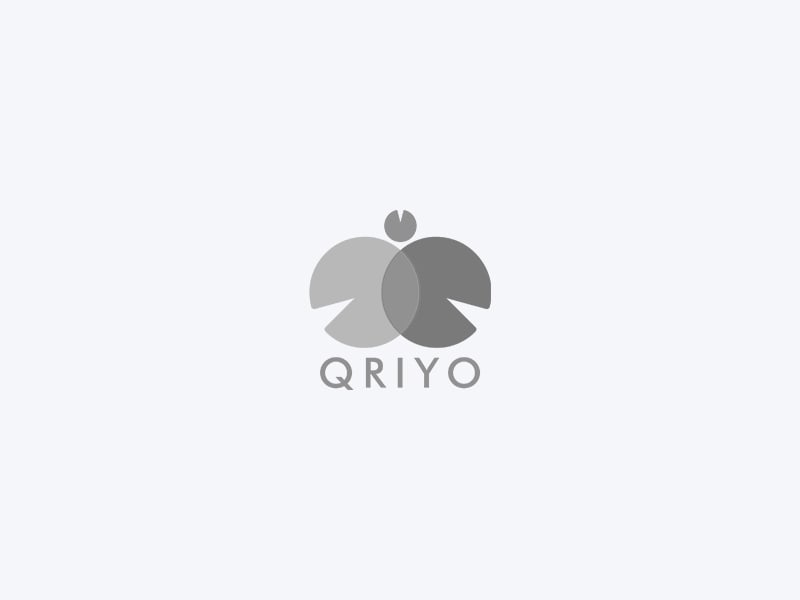Maths for Competitive Exam CAT, MAT, GMAT, CPTOverview
Maths is a fear, almost every student is scared of maths. And when it comes to big competitive exams, everyone wants a MATHS TUITION FOR CAT, MAT AND GMAT for helping the student with its concepts. get a maths tuition for Number systems, geometry, trigonometry, probability, permutation combination, algebra, mensuration, time and work, averages, percentages, profit and loss, quadratic and linear equations, etc.

Related Courses

Showing courses related to

Top Tutors for Maths for Competitive Exam CAT, MAT, GMAT, CPT

•Best Curated
Tutor for You
•Guaranteed Refund within
48 hours*
•Chapterwise Practice
Questions